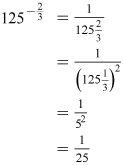Mathematics for science and technology

Start this free course now. Just create an account and sign in. Enrol and complete the course for a free statement of participation or digital badge if available.

Free course

# 1.5 The power of zero

At first sight a power of zero wouldn’t seem to mean anything. However, by using Rule 2 (dividing with powers) you can show that anything to the power zero is 1.

Clearly 2 ÷ 2 = 1.

However, using Rule 3, and the fact that 2 = 21, you can re-write this as:

21 ÷ 21 = 2(1–1) = 20

Showing that 20 = 1

This can be generalised to give: a0 = 1.

Note that you can now explain why .

By writing an as a(0 − n)

and, using Rule 2, this is the same as a0 ÷ an.

Since a0 = 1 this is then the same as:

In the same way, you can show that

can be written as 1 ÷ an

So, using Rule 2 and a0 = 1 gives:

Now try some examples.

## Activity 1 Working with power rules

Simplify each of the following examples. For a) and b) do this without a calculator initially.

• a.
• b.
• c.
• d.
• e.
• f.

### Discussion

• a. which can be written as
• b.• c.
• d.
• e.
• f.

Although you may arrive at the answer to an activity in a different way from that given here (because the rules of indices can often be applied in a different order), you should always get the same final answer.

All the examples you have looked at in this section have a simple answer but this will not always be the case, particularly where the calculation relates to a situation modelled on the real world.

In the next section you will be able to put these ideas in to practice again, as you learn about how to use powers to represent very small and very large numbers.

MST_1